• 如何可以让表格居中打印出来呢？。。    方法：页面布局--页面设置（右下角小箭头）--页边距-居中方式（在这里可以根据个人需要来设置《水平或垂直前面打对号》）然后打印就可以把表格居中打印在纸张上了。   ...
 做出的表打印出来一般都是偏向左上角。如何可以让表格居中打印出来呢？。。

方法：页面布局--页面设置（右下角小箭头）--页边距-居中方式（在这里可以根据个人需要来设置《水平或垂直前面打对号》）然后打印就可以把表格居中打印在纸张上了。

poi代码：
sheet.setHorizontallyCenter(true);//设置打印页面为水平居中

poi打印参考代码：

HSSFPrintSetup ps = sheet.getPrintSetup();
ps.setLandscape(true); // 打印方向，true：横向，false：纵向
ps.setPaperSize(PrintSetup.A4_PAPERSIZE); //纸张
sheet.setMargin(Sheet.BottomMargin,( double ) 0.5 );// 页边距（下）
sheet.setMargin(Sheet.LeftMargin,( double ) 0.1 );// 页边距（左）
sheet.setMargin(Sheet.RightMargin,( double ) 0.1 );// 页边距（右）
sheet.setMargin(Sheet.TopMargin,( double ) 0.5 );// 页边距（上）
sheet.setHorizontallyCenter(true);//设置打印页面为水平居中
sheet.setVerticallyCenter(true);//设置打印页面为垂直居中


展开全文• Excel 表格如何设置时间提前提醒，并且变化颜色excel表格设置了到期时间，如何让到期时间到期时，自动变成红色提示了？操作过程新的改变功能快捷键合理的创建标题，有助于目录的生成如何改变文本的样式插入链接与...
Excel 表格如何设置时间提前提醒，并且变化颜色excel表格设置了到期时间，如何让到期时间到期时，自动变成红色提示了？操作过程
excel表格设置了到期时间，如何让到期时间到期时，自动变成红色提示了？
操作过程
1、打开Excel，要实现功能的单元格或者列，点击开始——条件格式——管理规则。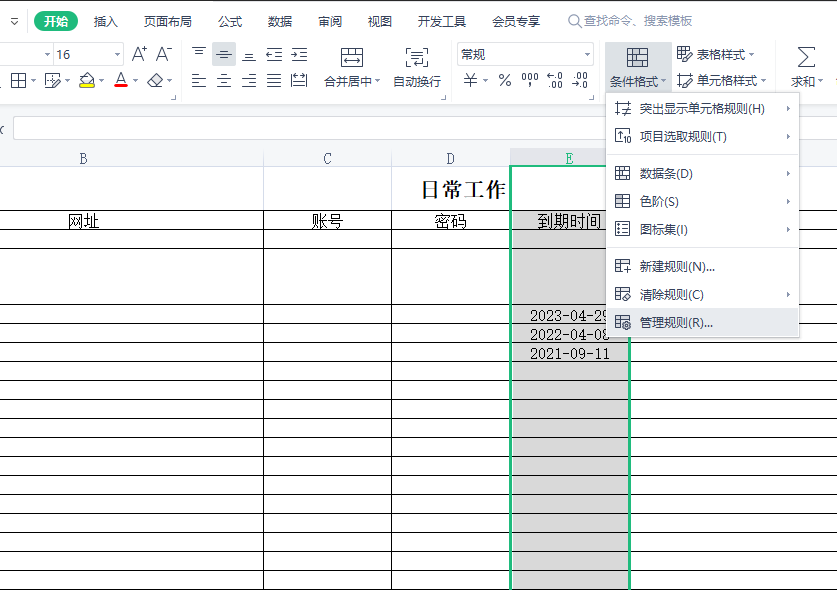2、规则如下。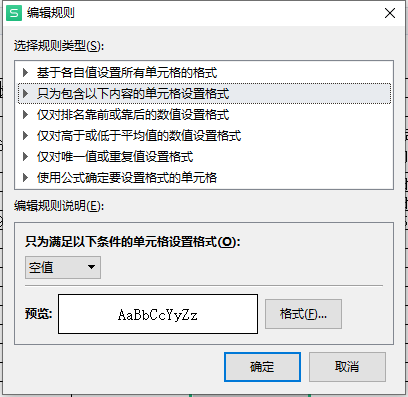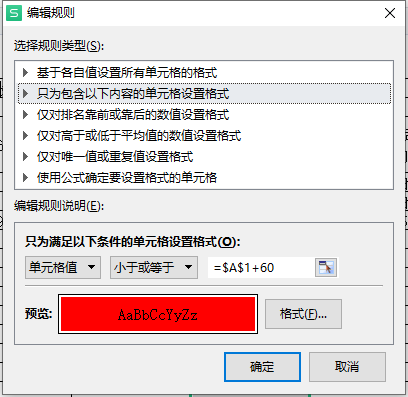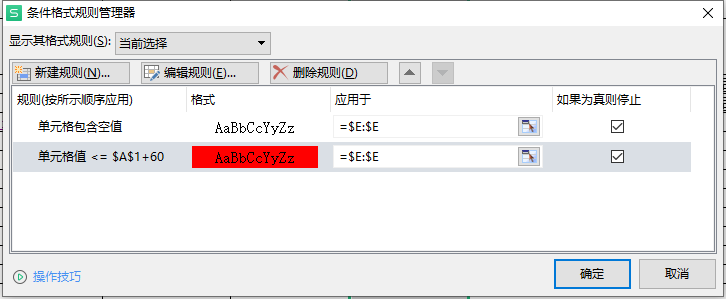3、这样就设置完毕了，我这里设置的是临近60天就描红。
4、实例：


展开全文• 1、快速对齐当数据对齐之后可以让表格更加的美观，我们一般设置的原则是：汉字字母列左对齐、数值列右对齐，对齐的方式很简单选中相应的区域，点击开始—对齐方式—左/右对齐 即可这时候给大家推荐一个小技巧：名字...
1、快速对齐当数据对齐之后可以让表格更加的美观，我们一般设置的原则是：汉字字母列左对齐、数值列右对齐，对齐的方式很简单选中相应的区域，点击开始—对齐方式—左/右对齐 即可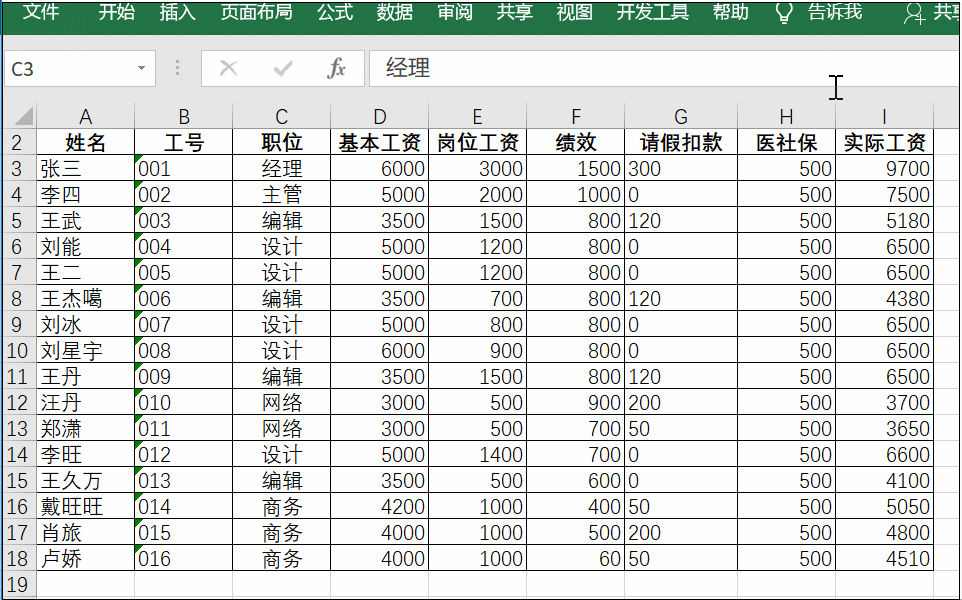这时候给大家推荐一个小技巧：名字如何对齐输入数据的时候，我们会遇到有的名字是两个的，有的是三个甚至四个，这时该如何对齐姓名呢？选中姓名一列按ctrl+1打开设置单元格对话框点击对齐——水平对齐——分散对齐 即可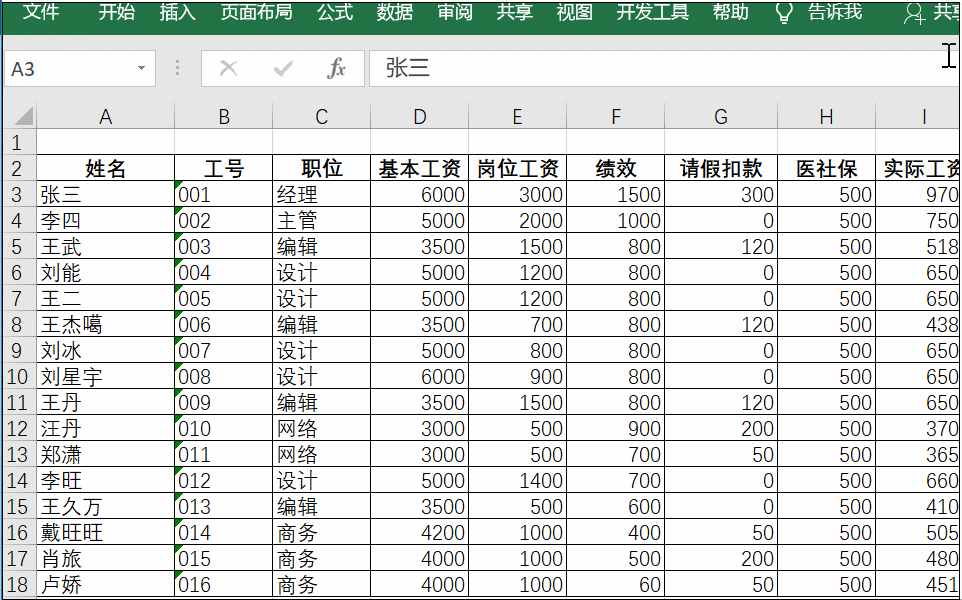表头居中如果想将表头设置为居中位置，一般情况下会先合并区域，之后再将文本内容设置居中，这样往往会影响后期表格的处理，使一些数据发生混乱，这时候其实可以直接选中区域内容，点击开始——对齐方式——跨列居中，这样就不会影响后期处理。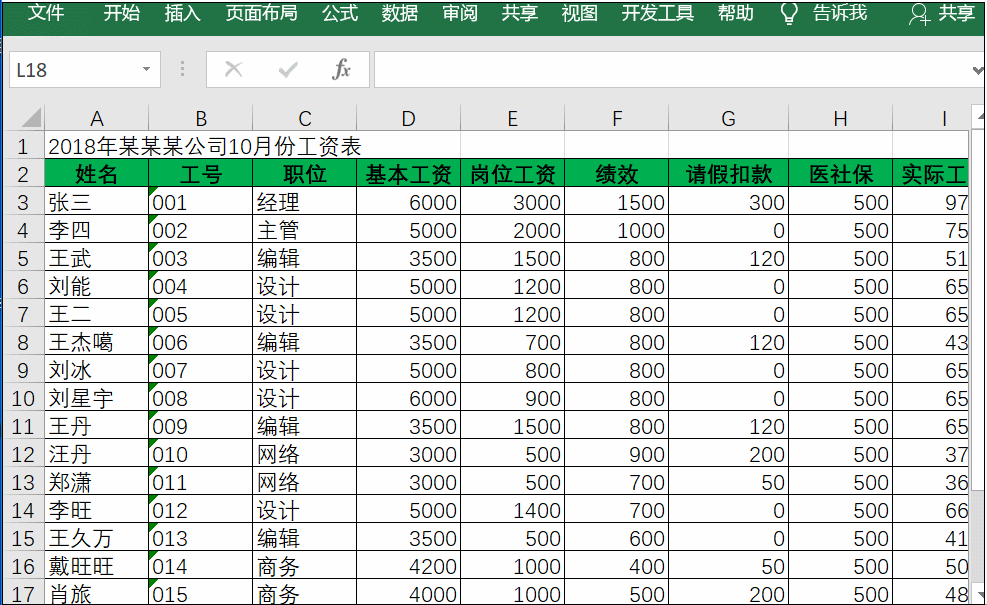2、行列调整我们一般会将一些编号和日期放在首列，如果遇到不在首列的情况下，这时候直接可以拖动进行整理首先选中列，当鼠标变为是十字架后按住Shift键不放就可以移动位置。移动到所需位置松开即可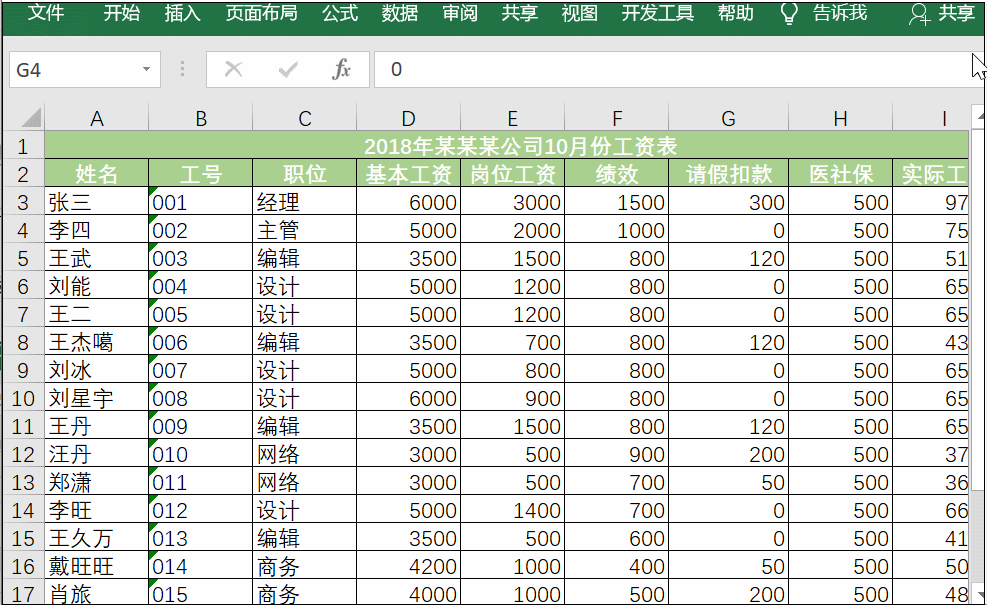3、边框设置EXCEL自带的网格线默认黑色单线，如果想要更改颜色以及线的样式，这时可以这样做！可以通过视图，取消网格线再次选中区域，点击开始——边框——其他边框，在相应的对话框中设置好后相应的直线样式和颜色，最后点击确定即可。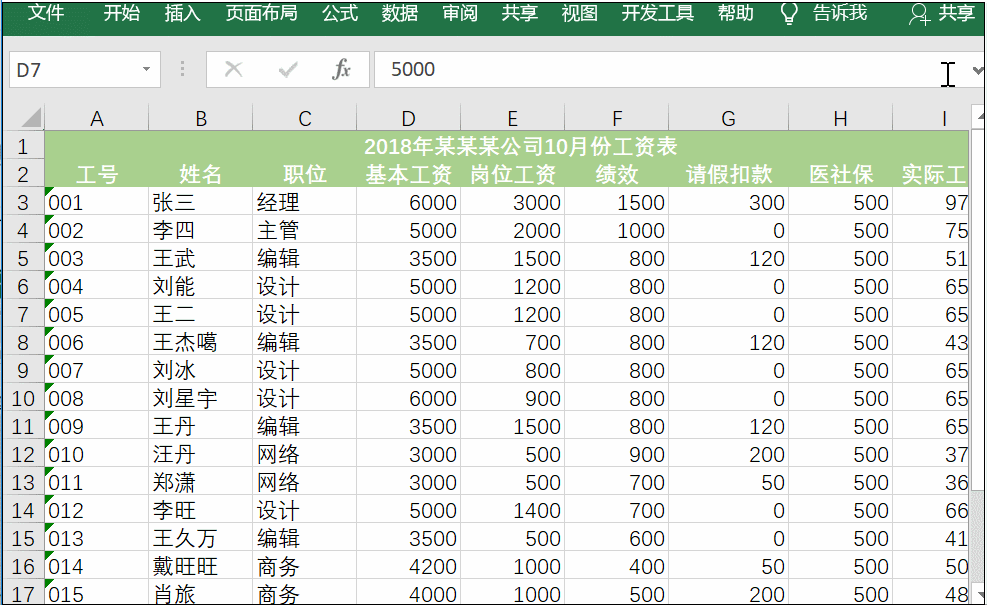4、条形图用法为了更好的查看数据，可以在表格中制作一个小图表，这时可以选中数据点击开始——条件格式——条形图。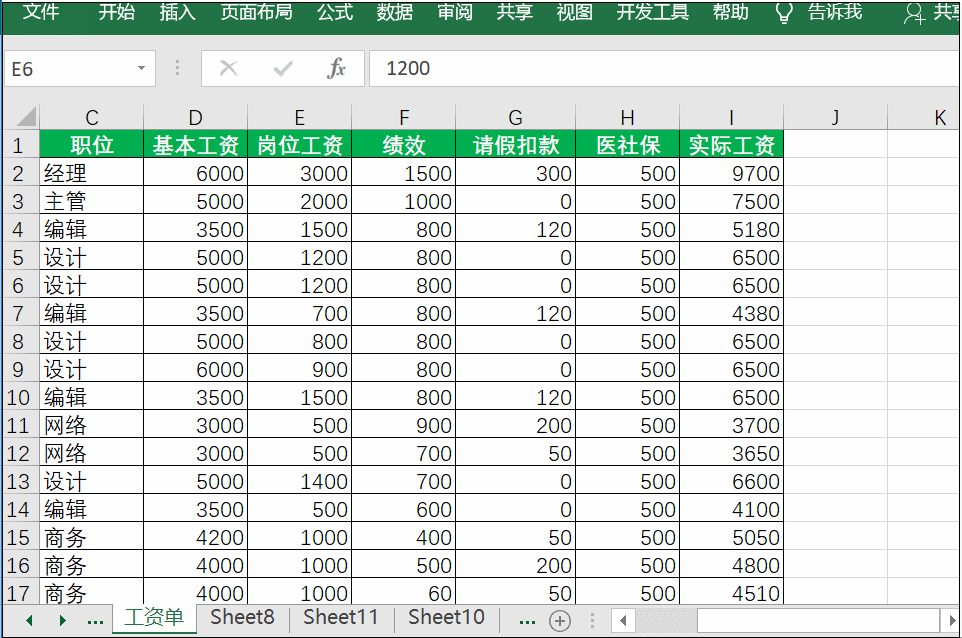展开全文• 我在怎样合并单元格里的字居中，怎样改变字体。 请不吝赐教。。。 ========================================================== 首先在VB中引用microsoft excel object library 假设c盘根目录下...
如何通过VB合并Excel单元格以及设置Excel行高？

例如：我想把第一列的第4，5，6，7行合并。。。我在怎样让合并单元格里的字居中，怎样改变字体。 请不吝赐教。。。

==========================================================

首先在VB中引用microsoft excel object library

假设c盘根目录下有个1.xls的文件
…………

问题补充：

你的要求很简单，

一行指令恐怕不行，

最少要三行

代码如下：

Dim xlApp As Excel.Application

Dim xlBook As Excel.Workbook

Dim xlSheet As Excel.Worksheet

Dim i As Integer

Set xlApp = CreateObject("Excel.Application")

Set xlBook = xlApp.Workbooks.Open("C:\1.xls")

Set xlSheet = xlBook.Worksheets(1) '引用第1张工作表

For i = 3 To 100 Step 3 '想到哪行就把100改成几

xlSheet.Range(Cells(i, 1), Cells(i, 2)).Merge '合并a1:b2单元格

Next

xlSheet.Rows(10).RowHeight = 100 '把第10行高度设为100

xlBook.Save

xlApp.Application.Quit

Set xlApp = Nothing '表忘释放引用

End Sub

运行一下，看看满足要求否。

===========================================================================================

Sub Macro1()

Range("A4:A7").Select
With Selection
.HorizontalAlignment = xlCenter
.VerticalAlignment = xlCenter
.WrapText = False
.Orientation = 0
.IndentLevel = 0
.ShrinkToFit = False
.MergeCells = False
End With
Selection.Merge
With Selection.Font
.Name = "隶书"
.FontStyle = "常规"
.Size = 12
.Strikethrough = False
.Superscript = False
.Subscript = False
.OutlineFont = False
.Underline = xlUnderlineStyleNone
.ColorIndex = xlAutomatic
End With
End Sub

=========================================================================================

VB创建Excel表格，合并单元格，生成图形等操作

Private Sub Command4_Click()
On Error Resume Next
''''''''''''''''''''''''''''''''''''''''''''''''''''''''Create Excel Table''''''''''''''''''''''''''''''''''''''''''
Dim xlApp As Excel.Application
Dim xlBook As Excel.Workbook
Dim xlSheet As Excel.Worksheet
Dim xlSheet1 As Excel.Worksheet
Dim i As Integer, tmHour As String
''''''''''''''''''''''''''''''''''''''''''''''''''''''''''''
On Error Resume Next

''''''''''''''''''''''''''''''''''''''''''''''''''''''''''
Set xlApp = Excel.Application
'xlBook.Activate
Set xlSheet = xlBook.Worksheets(1) '''''''''''''''''''''''''''''''''引用第1张工作表
xlApp.ActiveSheet.Rows.VerticalAlignment = xlVAlignCenter '''''垂直方向居中
xlApp.ActiveSheet.Rows.HorizontalAlignment = xlVAlignCenter '''水平方向居中
xlSheet.Name = "实测值"
Set xlSheet1 = xlBook.Worksheets(2)
xlSheet1.Name = "Chart"
With xlSheet
For i = 2 To 11
.Range(Cells(1, 1), Cells(1, i)).Merge ''''''''''''''''''''合并A-K单元格
Next
' .Cells(1, 1).ForeColor = RGB(100, 150, 255)
.Cells(1, 1).Font.Size = 25

''''''''''''''''''''''''''设置行高'设置列宽
For i = 1 To 22
.Rows(i).RowHeight = 25
Next
For i = 1 To 11
.Columns(i).ColumnWidth = 15
Next
'''''''''''''''''''''''''''合并单元格
For i = 3 To 22

If i < 8 Then
.Range(Cells(3, 1), Cells(i, 1)).Merge '''''''''''合并A3-A7单元格
.Range(Cells(3, 8), Cells(i, 8)).Merge '''''''''''合并H3-H7单元格
ElseIf i < 13 Then
.Range(Cells(8, 1), Cells(i, 1)).Merge
.Range(Cells(8, 8), Cells(i, 8)).Merge
ElseIf i < 18 Then
.Range(Cells(13, 1), Cells(i, 1)).Merge
.Range(Cells(13, 8), Cells(i, 8)).Merge
ElseIf i < 23 Then
.Range(Cells(18, 1), Cells(i, 1)).Merge
.Range(Cells(18, 8), Cells(i, 8)).Merge
End If
Next
''''''''''''''''''''''''''''''''''''''''''''
.Range("A1", "K22").Borders.LineStyle = xlContinuous '''''''单元格边框
.Range("A1", "K22").Borders.Color = vbBlue '''''''''''''''''边框颜色
.Range("A1", "K22").Interior.Color = RGB(100, 180, 0) ''''''区域 背景色

''''''''''''''''''''''''''''''
.Range("A1").Value = "iWatt 项目"
.Range("A1").Font.Color = vbRed ''''''''''''''设置字体颜色
.Range("A1").Font.Name = "楷书" ''''''''''''''设置字体字型
.Range("A1").Font.Size = 30 ''''''''''''''''''设置字体字号
'''''''''''''''''''''''''''''''''''''''
.Range("A2").Value = "输入电压(VAC)"
.Range("B2").Value = "输入功率(W)"
.Range("C2").Value = "输出电压(V)"
.Range("D2").Value = "输出电流mA)"
.Range("E2").Value = "输出功率(W)"
.Range("F2").Value = "纹波电压(A)"
.Range("G2").Value = "效率(%)"
.Range("H2").Value = "过流点(A)"
.Range("I2").Value = "初级到次级功率损耗(W)"
.Range("J2").Value = "平均功率%"
.Range("K2").Value = "需符合CEC标准"
'''''''''''''''''''''''''''''''''''电压值
.Range("A3").Value = "90"
.Range("A8").Value = "115"
.Range("A13").Value = "230"
.Range("A18").Value = "264"
'''''''''''''''''''''''''''''''''''负载值

.Range("D3").Value = "0"
.Range("D8").Value = "0"
.Range("D13").Value = "0"
.Range("D18").Value = "0"
End With
tmHour = "-" & Hour(Time)
tmHour = tmHour & "-" & Minute(Time)
tmHour = tmHour & "-" & Second(Time)
xlApp.ActiveWorkbook.SaveAs App.Path & "\" & Format(Date, dddd, mmmm, yyyy) & tmHour + ".xls"
xlApp.Workbooks.Close
xlApp.Quit
Set xlApp = Nothing '释放引用
''''''''''''''''''''''''''''''''''''''''''''''''''''''''''''''''''''''''''''写入数据'''''''''''''''''''''''''''''''''''''''''''''
Dim j, LengthTXT, k, Num, NEXCEL As Integer
Dim StrTxt As String
On Error Resume Next
'''''''''''''''''''''''''''计算数组的围数 NUM
LengthTXT = Len(Text1.Text)
StrTxt = Text1.Text
Num = 1
For i = 1 To LengthTXT
If Mid(Text1.Text, i, 1) = "," Then
Num = Num + 1
End If
Next
ReDim StrDataArray(Num)  '重定义围数
'''''''''''''''''''''''''''''''''''''''''''
''''''''''''''''''''''''''''''赋值给数组 StrDataArray
If Num = 1 Then
StrDataArray(Num) = StrTxt
Else
For i = 1 To LengthTXT
StrData = StrData & Mid(StrTxt, i, 1)
k = k + 1
If Mid(StrTxt, i, 1) = "," Then
j = j + 1
StrDataArray(j) = Left(StrData, k - 1)
StrData = ""
k = 0
End If
StrDataArray(Num) = StrData
Next
End If
'    '''''''''''''''''''''''''''''check StrDataArray(i)
'    For i = 1 To Num
'    MsgBox StrDataArray(i) & "  " & i
'    Next
'''''''''''''''''''''''''''''''''''数值分段存储到数组,每组为一个实测值
Dim TowArray() As String
Dim WS, N As Integer
WS = Num \ 4 '''''''''''''''''围数
ReDim TowArray(WS, 4)
For i = 1 To Num - 2
N = i \ 4
For j = 1 To 4
'If i \ 4 = 0 Then
TowArray(N + 1, j) = StrDataArray(j + 4 * N)
' End If
Next
Next

'    ''''''''''''''''''''''''''''''''check  TowArray(N + 1, j)
'    For i = 1 To WS
'      MsgBox TowArray(i, 1) & TowArray(i, 2) & TowArray(i, 3) & TowArray(i, 4)
'    Next
'    ''''''''''''''''''''''''''''''''''数值转换
''''第4个字节转换为2进制

ReDim ByteDataString(WS)
For i = 1 To Num \ 4
'MsgBox TowArray(i, 4) MsgBox CStr(TowArray(i, 4))
ByteDataString(i) = HexToByte(CStr(TowArray(i, 4))) '''''转换为2进制,8位
'MsgBox ByteDataString(i) & " " & i
Next
'''''''''''''''''''''''''''''''''''''''''''''''

''''''''''''''''打开Excel 文件！
Dim filename As String
With CommonDialog1
.DialogTitle = "打开Excel文件"

.Filter = "(Excel)*.xls| *.xls"
.ShowOpen
filename = .filename
' MsgBox filename
End With
'''''''''''''''''''''''''
Dim xllApp As Excel.Application
Dim xllBook As Excel.Workbook
Dim xllSheet As Excel.Worksheet
Dim xllSheet1 As Excel.Worksheet
Dim StrRow As String
'Dim i As Integer
Set xllApp = CreateObject("Excel.Application")
Set xllBook = xllApp.Workbooks.Open(filename)
Set xllSheet = xllBook.Worksheets(1) '引用第1张工作表
Set xllSheet1 = xllBook.Worksheets(2)
'''''''''''''''''''''''''''''''''''''''''''''''''''''''''将数据写入到Excel单元格中

With xllSheet
For i = 1 To WS
NEXCEL = i
StrRow = "B" & CStr(i + 2)
' MsgBox ByteDataString(i) '& StrRow
.Range(StrRow).Value = ValueOfData(ByteDataString(i), NEXCEL) '''''设置一个返回函数
Next
End With
Set ct = xllApp.Worksheets("Chart").ChartObjects.Add(100, 40, 300, 350) '插入图形''位置（10，40）为图形位置，（220，120）为图形的大小
ct.Chart.ChartType = xlLineStacked 'xlColumnClustered '块状图 'xl3DColumnStacked '立體直條圖'xl3DPie '图形类型为饼图
ct.Chart.SetSourceData Source:=Sheets("实测值").Range("B3:B6"), PlotBy:=xlColumns
With ct.Chart
.HasTitle = True
.ChartTitle.Characters.Font.Size = 20
.ChartTitle.Characters.Text = "折线图" '图表标题为饼图
End With
ct.Chart.ApplyDataLabels 2, True '标志旁附图例项标志***  标志数值
xllBook.Save
xllApp.ActiveWorkbook.Save
xllApp.Application.Quit
Set xllApp = Nothing '表忘释放引用
End Sub
展开全文• 私信回复关键词【学一招】~一次性获取Excel小技巧合集！助你成为高效职场人！大家好，我是秋小 E~这里是秋叶 Excel 的【问答精华】专栏！问题主要来自秋叶 Excel 学习班的同学，回答由讲师、助教共同...01如何居中...
• 使用Excel录入数据的过程中，我们总会发现一些相同的内容重复录入，为了让表格内容看起来更加清晰，对于相同内容的单元格，我们可以进行合并，但如何批量合并呢？今天跟大家分享2个方法：分类汇总和数据透视表。方法...
• 用Excel打印文件仍然是一个令人头痛的问题，想当初，我就不知道打印前要先设置，直接预览打印，不知道浪费了老板多少张纸，幸好它们后来还是有用的，摇身一变成为草稿纸~今天就通过6个Excel表格打印小技巧，大家...
• 对于许多朋友来说，用Excel打印文件仍然是一个头条的问题，文件如何表格中进行打印设置许多朋友还是比较陌生。今天我们就通过13个打印小技巧，大家了解一下打印机的常规操作。1、打印居中显示更改居中方式，使...
• 有助于目录的生成如何改变文本的样式插入链接与图片如何插入一段漂亮的代码片生成一个适合你的列表创建一个表格设定内容居中、居左、居右SmartyPants创建一个自定义列表如何创建一个注脚注释也是必不可少的KaTeX数学...python pandas 数据分析 数据挖掘
• 私信回复关键词【学一招】~一次性获取Excel小技巧合集！助你成为高效职场人！大家好，我是秋小 E~这里是秋叶 Excel 的【问答精华】专栏！问题主要来自秋叶 Excel 学习班的同学，回答由讲师、助教共同...01如何居中...
• PHPExcel的强大之处就是可以我这样不会excel程序员，也能生成好看的表格，绘制合适的图表。 本篇文章重点是如何绘制图表和组合图表。因为优化表格在网上有很多中文文档，所以这里没必要再重复。但是我会结合使用...PHP PHPExcel 组合图 中文手册
• 对于许多朋友来说，用Excel打印文件仍然是一个头条的问题，文件如何表格中进行打印设置许多朋友还是比较陌生。今天我们就通过13个打印小技巧，大家了解一下打印机的常规操作。1、打印居中显示更改居中方式，使...
• 1、打印表格如何居中如图所示，打印表格时，发现表格位置在左上角，如何让打印出来的表格居中显示呢？点击页面布局——页面设置，在打开的界面中选择页边距，随后在居中方式中，勾选水平和垂直复选框，点击确定即可...
• 同时在Windows和macOS系统下演示Excel的使用方法 本系列课程使用Excel2016讲解，目的是学员掌握如何充分利用Excel强大的功能高效办公，本课程的主要内容如下： 用12步做出老板满意的表格 尽量不要直接隐藏行和列...wps office office wps
• 　使用宏功能，可以我们在处理电子表格的时候，仅通过一步的操作，就能实现需要通过多步才能完成的操作。 下面，我们来学习一下，如何Excel中使用自定义宏。 　要使用自定义宏，分为两步，一为自制宏；二...
• ## EXCEL函数公式集

热门讨论 2010-03-16 03:26:38
如何让EXCEL自动从头统计到当前单元格 统计人数 统计人数 求各分数段人数 有什么方法统计偶数 如何显示 工资统计中的问题 统计数据问题一例 根据给定的条件，对数据进行合计 十列数据合计成一列 统计汉字字符个数 ...
• 用python预处理excel表格数据，见下方链接，亲测可用 链接: link. 图片: 带尺寸的图片: 居中的图片: 居中并且带尺寸的图片: 当然，我们为了用户更加便捷，我们增加了图片拖拽功能。 如何插入一段漂亮的代码片 ...python csv
• 这里写自定义目录标题原理新的改变功能快捷键合理的创建标题，有助于目录的...在Excel表格中，引用单元格（包括引用其他工作簿）的数据时，==“显示公式”==的功能可以让Excel直接显示公式和所引用的单元格地址，然后我
• 如何让EXCEL自动从头统计到当前单元格 统计人数 统计人数 求各分数段人数 有什么方法统计偶数 如何显示 工资统计中的问题 统计数据问题一例 根据给定的条件，对数据进行合计 十列数据合计成一列 统计汉字字符个数 ...
• 辛辛苦苦做出一个Excel文档，老板打印出来。老板：你怎么不能放在一页？...1、打印页面页数设置Excel表格在打印时常常由于表格太大，不能在一页中完全打印出来，或者因为太长，不知如何分页。这里教大家一...
• 这时，再选中B2格，光标指向B2矩形右下角的“■”，当光标变成"＋"时按住光标沿B列拖动到适当的位置即完成函数值的计算。图７绘制曲线：点击工具栏上的“图表向导”按钮，选择“X，Y散点图”（如图7），然后在出现...
• 职场人士必学的10种Excel打印技巧及详细的操作说明 Excel文件打印是我们每天工作...Excel默认打印靠纸的左边打印，如果我们制作表格比较窄，打印出来在纸的左边就显得不是很好看，下面是如何调整居中打印的方法。 方法经验分享 数据分析
• 对于许多朋友来说，用Excel打印文件仍然是一个头条的问题，文件如何表格中进行打印设置许多朋友还是比较陌生。今天我们就通过13个打印小技巧，大家了解一下打印机的常规操作。1、打印居中显示更改居中方式，使...
• 4) 如何让dbgrideh1 显示数据时只显示两位小数...................................................30 5) 获得当前DBGridEh表中单元格的序号.............................................................30 6) ...
• 实例058　CSS控制垂直居中 实例059　CSS实现的图文混排 3.2　表格样式 实例060　只有外边框的表格 实例061　彩色外边框的表格 实例062　单元格的边框变色 实例063　表格外边框具有霓虹灯效果 实例064　控制表格指定...Java web
• 实例058　CSS控制垂直居中 实例059　CSS实现的图文混排 3.2　表格样式 实例060　只有外边框的表格 实例061　彩色外边框的表格 实例062　单元格的边框变色 实例063　表格外边框具有霓虹灯效果 实例064　控制表格指定...Java web
• ## word使用技巧大全

热门讨论 2011-03-18 20:37:53
方法3：如何设置居中的公式 61 三十一、拼音位置 62 三十二、密码破解 62 1、破解Word密码不是梦 62 2、Excel也别跑 63 三十三、PDF转DOC 64 三十四、解密高手 64 三十五、公式宽度 66 全部一次调整 67 局部调整方法...
• 问题44 如何让切换按钮定时弹起 481 问题45 如何在菜单栏上创建万年历 482 问题46 如何生成旋转的转盘 483 第9章 串口编程 486 9.1 知识点归纳 486 9.1.1 串口概述 486 9.1.2 串口对象的属性 488 9.1.3 串口...